# How to Generate Lottery Numbers in Excel?

Generating lottery numbers by using Excel is an easy and time-saving process. The described task can be done by using two different examples. The first practice involves using the VBA code. The user needs to create a module and execute the module to generate the lottery number. The second example is based on the use of kutools. In the kutools example, the user is required to use a random value function to implement the required functionality. Since the lottery number should be an integer data. Therefore, this tutorial is generating the lottery number with an integer type.

## To generate the lottery number in Excel

### Example 1

#### Step 1

Consider the below-provided workbook. This workbook contains a column entry “Lottery number”.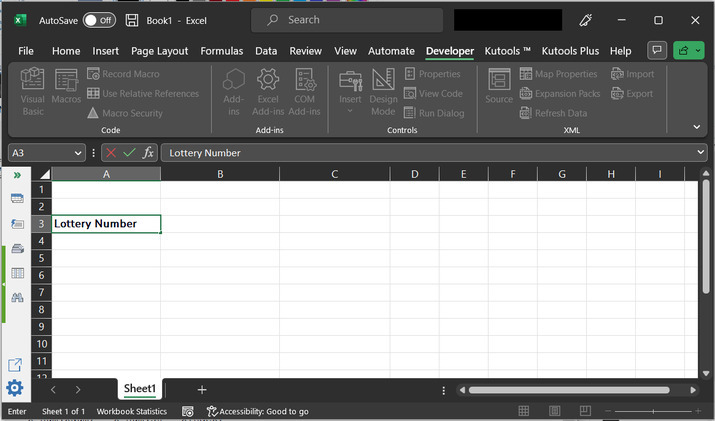#### Step 2

After that go to the “Developer” tab and then go to the “Code” section and choose the “Visual Basic” option.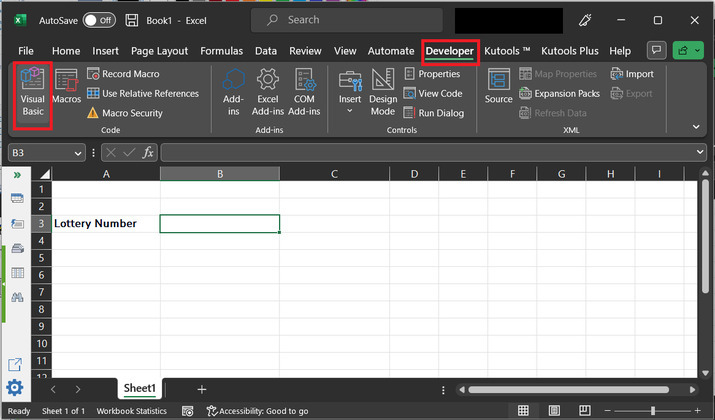#### Step 3:

The above taken steps will open the below given code editor.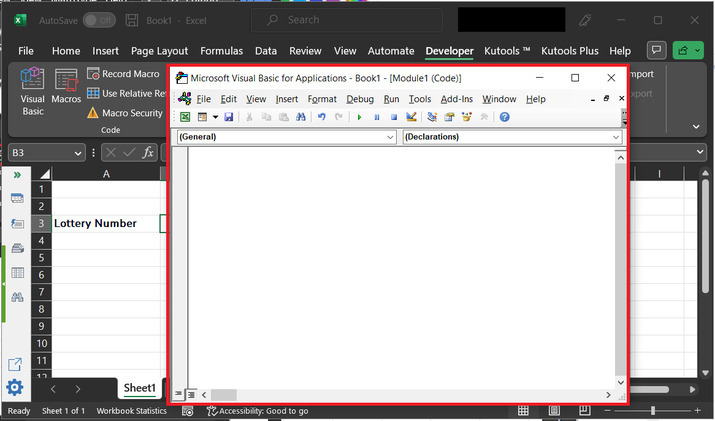#### Step 4

In the above code area, type below given code.

#### Code

' define generate_lottery_code
Sub Generate_Lottey_Code()
' declaring range and integer
Dim ran_ge As Range
Dim wrk_rng As Range
Dim x_num(49) As Integer
On Error Resume Next
' setting title dilog box heading
Title_Id = "Input cell to display data value"
' setting selection range
Set wrk_rng = Application.Selection
' asking user to enter input value
Set wrk_rng = wrk_rng.Range("A1")
' for loop
For xIndex = 1 To 49
'set value to xIndex
x_num(xIndex) = xIndex
'next instruction
Next
For xIndex = 1 To 6
'rounding data value
xNum = 1 + Application.Round(Rnd * (49 - xIndex), 0)
'setting offset data
wrk_rng.Offset(0, xIndex - 1).Value = x_num(xNum)
' setting variable value
x_num(xNum) = x_num(50 - xIndex)
Next
' end of sub method
End Sub


## Consider code snapshot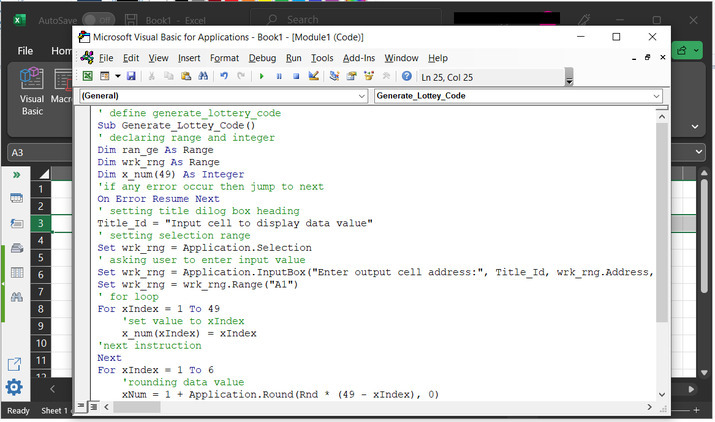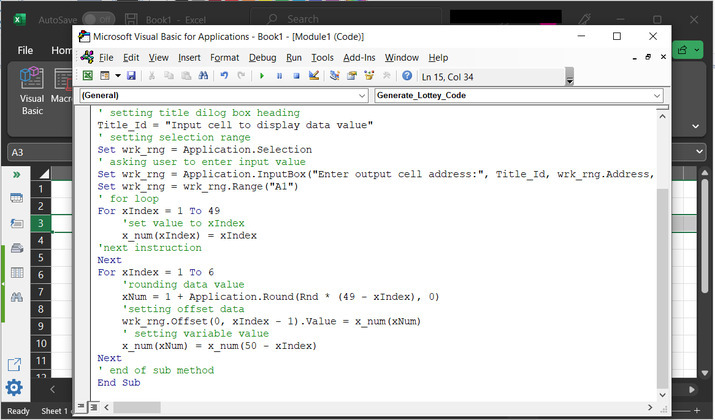#### Step 5:

After that click on “Run” to obtain the required output.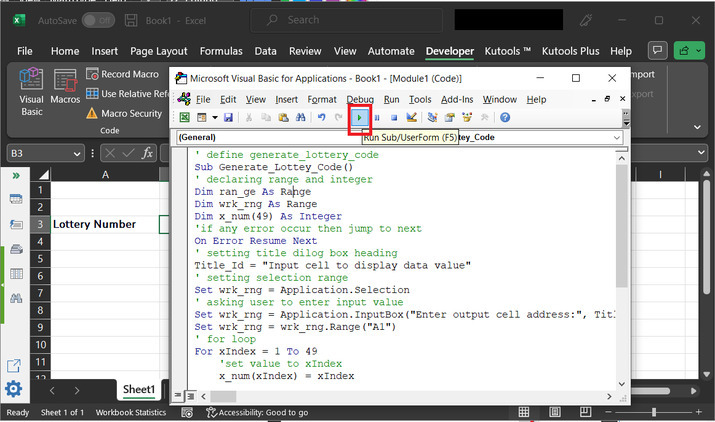#### Step 6

The above step will display the “Input cell to display data value” dialog box. In the appeared dialog box, type range to display results. For this case the taken cells are B3.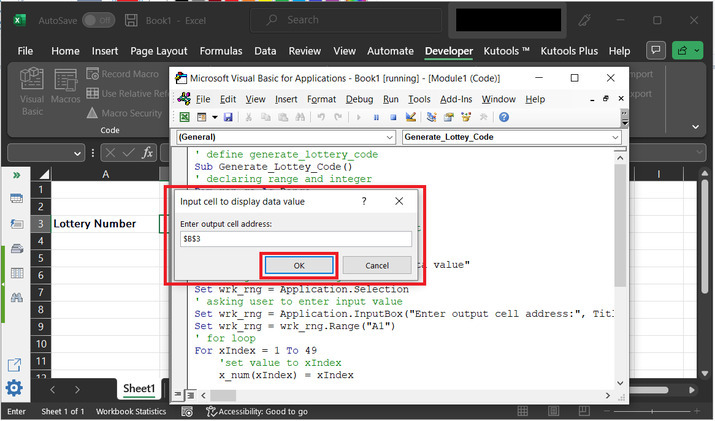#### Step 7

The generated output will be given below: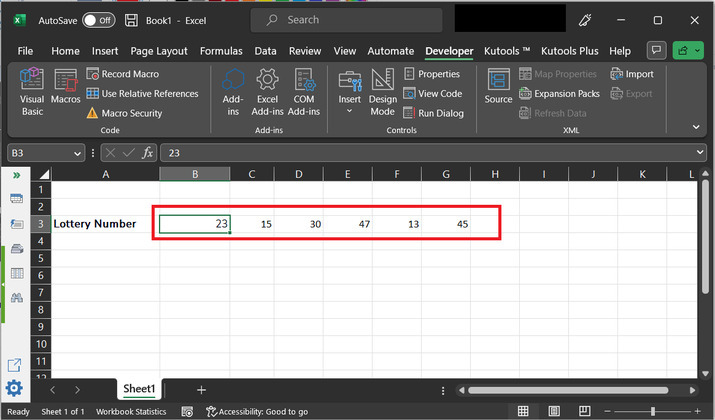## To generate lottery numbers in Excel by using the kutool:

### Example 2

#### Step 1

Consider the below-provided workbook. This workbook contains a column entry “Lottery number”.#### Step 2

Go to the “Kutool” tab and choose “Editing”. Under the “Editing” section, go to the “Insert” option, and then click on “Insert Random Data” as highlighted in the below image.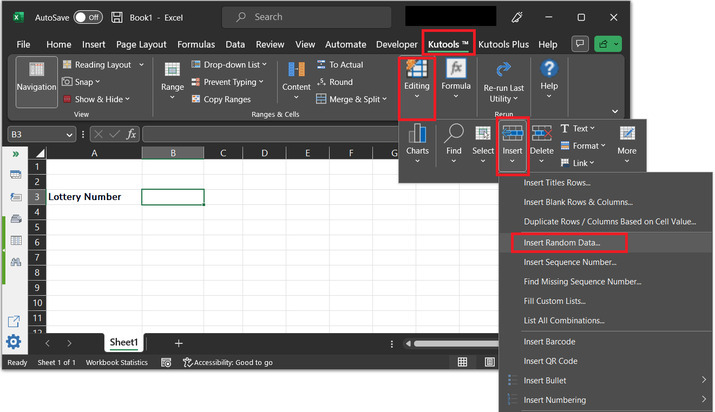#### Step 3

This will open the “Insert Random Data” dialog box. Inside the generated box, put the data range as 1 to 100, to generate the lottery number. After that tick the “Unique values” option. This will generate a unique random number. Finally, click on “Ok” button.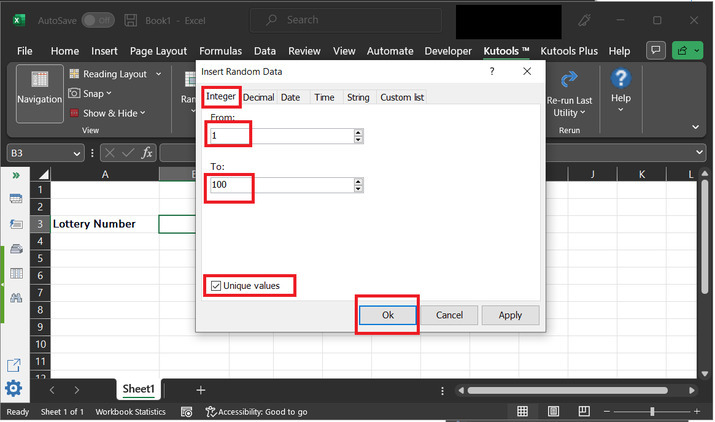#### Step 4

This will produce the below given results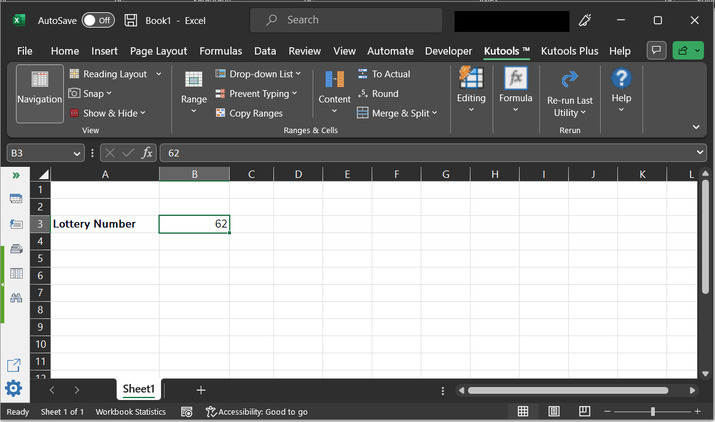## Conclusion

The article illustrates two examples to create the lottery number. After the successful completion of the above article, the user will able to understand the process of generating a unique lottery number by using kutools and VBA editor.

Updated on: 17-Apr-2023

121 Views